# 写在前面

CVPR作为计算机视觉领域的顶级会议（CCF-A），目前有4篇联邦学习相关的论文

1. Multi-Institutional Collaborations for Improving Deep Learning-Based Magnetic Resonance Image Reconstruction Using Federated Learning
2. Model-Contrastive Federated Learning
3. FedDG: Federated Domain Generalization on Medical Image Segmentation via Episodic Learning in Continuous Frequency Space
4. Soteria: Provable Defense Against Privacy Leakage in Federated Learning From Representation Perspective

# 一、Motivation

• 联邦学习的关键挑战是客户端之间数据的异质性（Non-IID），尽管已有很多方法（例如FedProx，SCAFFOLD）来解决这个问题，但是他们在图像数据集上的效果欠佳（见实验Table1）。
• 传统的对比学习是data-level的，本文改进了FedAvg的本地模型训练阶段，提出了model-level的联邦对比学习（Model-Contrastive Federated Learning）
• 作者从NT-Xent loss中获得灵感，提出了model-contrastive loss。model-contrastive loss可以从两方面影响本地模型 1. 本地模型能够学到接近于全局模型的representation 2. 本地模型可以学到比上一轮本地模型更好的representation

# 二、背景知识

## 对比学习SimCLR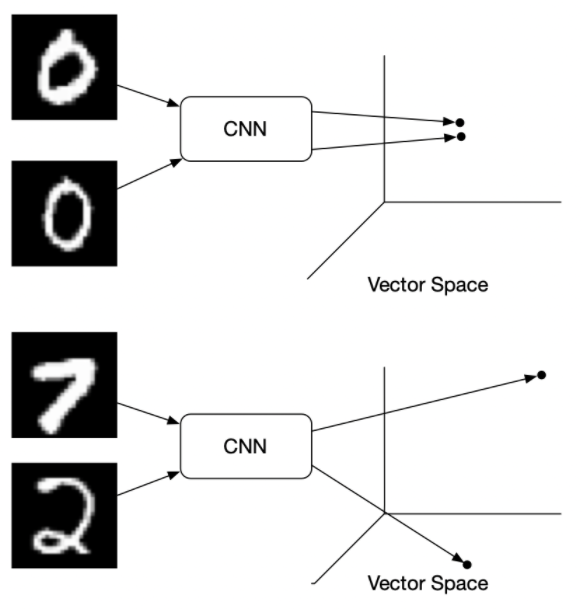SimCLR是对比学习中经典的方法。

$$l_{i, j}=-\log \frac{\exp \left(\operatorname{sim}\left(x_{i}, x_{j}\right) / \tau\right)}{\sum_{k=1}^{2 N} \mathbb{I}{[k \neq i]} \exp \left(\operatorname{sim}\left(x{i}, x_{k}\right) / \tau\right)}$$

SimCLR伪代码 paper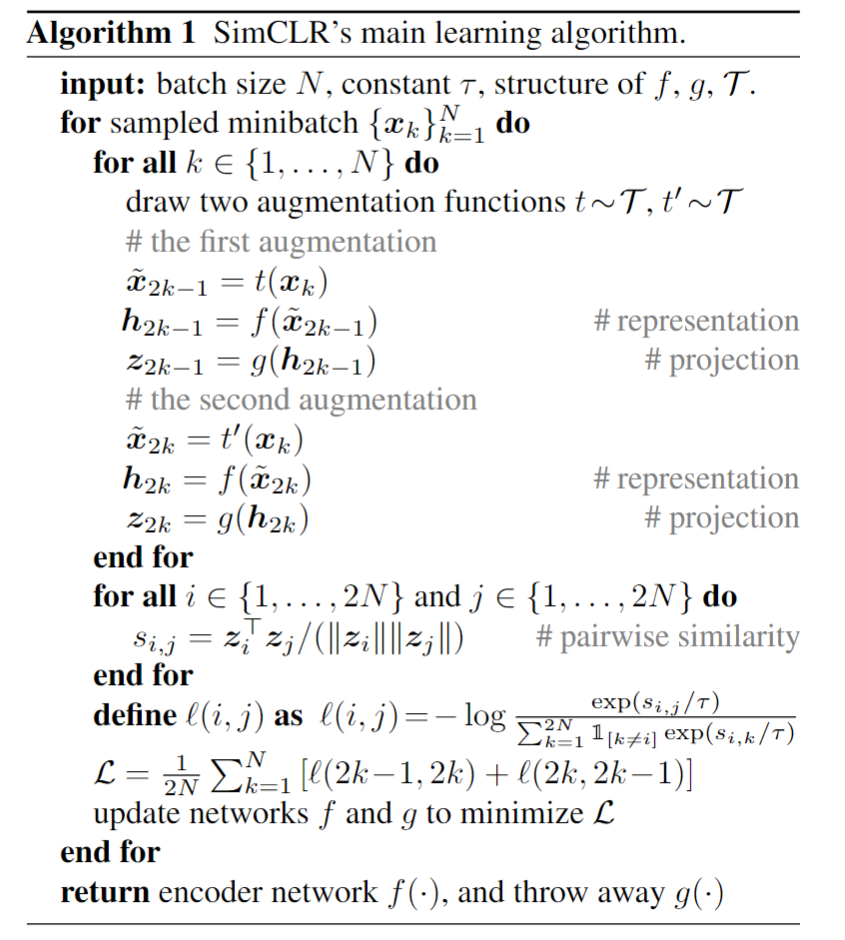## Preliminary Experiment

the model trained on the whole dataset is able to extract a better feature representation than the model trained on a skewed subset.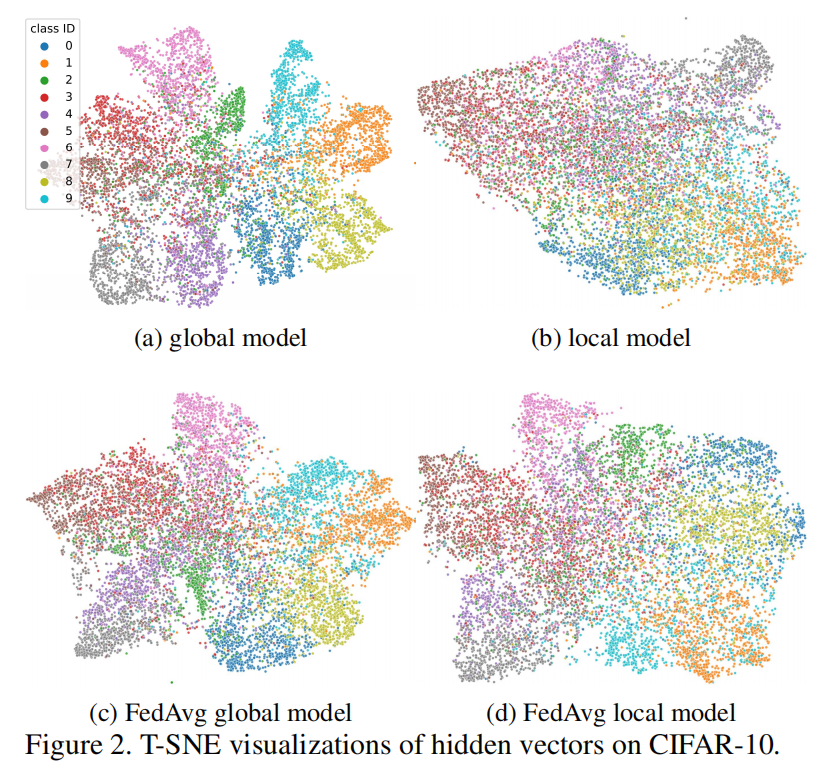• 2a：用所有数据集放在一起训练一个CNN模型。
• 2b：将所有数据集以Non-IID的方式划分10个客户端，各自训练CNN模型，最后随机选择一个客户端的模型。
• 2c：在10个客户端上使用FedAvg算法训练得到一个global model（10个本地模型加权平均）
• 2d：在10个客户端上使用FedAvg算法训练，然后随机选择一个客户端的local model。（2d学习到的蓝色的类别表征明显比2c差）

# 三、方法：MOON

## 问题定义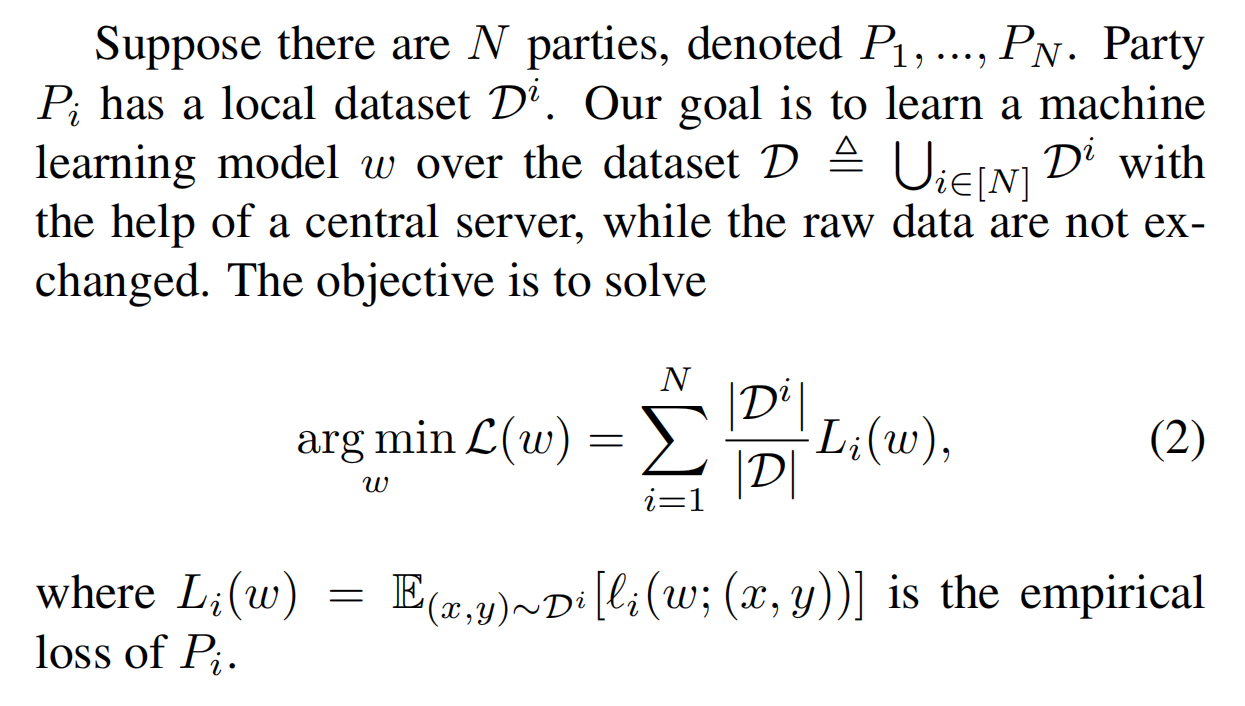## MOON的目标

Since there is always drift in local training and the global model learns a better representation than the local model, MOON aims to decrease the distance between the representation learned by the local model and the representation learned by the global model, and increase the distance between the representation learned by the local model and the representation learned by the previous local model.

## MOON的loss函数

MOON在本地训练阶段，会有三个表征（representation）

• $z_{prev}=R_{w_i^{t-1}}(x)$（上一轮本地训练好的发往server的模型得到的表征）固定
• $z_{\text {glob }}=R_{w^{t}}(x)$（这轮开始时发送到本地的全局模型得到的表征）固定
• $z=R_{w_i^{t}}(x)$ （这轮正在被更新的本地模型得到的表征）不断被更新

With model weight $w$，$R_w(·)$ to denote the network before the output layer (i.e., $R_w (x)$ is the mapped representation vector of input x).

$$\ell_{\text {con }}=-\log \frac{\exp \left(\operatorname{sim}\left(z, z_{\text {glob }}\right) / \tau\right)}{\exp \left(\operatorname{sim}\left(z, z_{\text {glob }}\right) / \tau\right)+\exp \left(\operatorname{sim}\left(z, z_{\text {prev }}\right) / \tau\right)}$$

MOON的优化目标（loss）如下：
$$\ell=\ell_{\text {sup }}\left(w_{i}^{t} ;(x, y)\right)+\mu \ell_{\text {con }}\left(w_{i}^{t} ; w_{i}^{t-1} ; w^{t} ; x\right)$$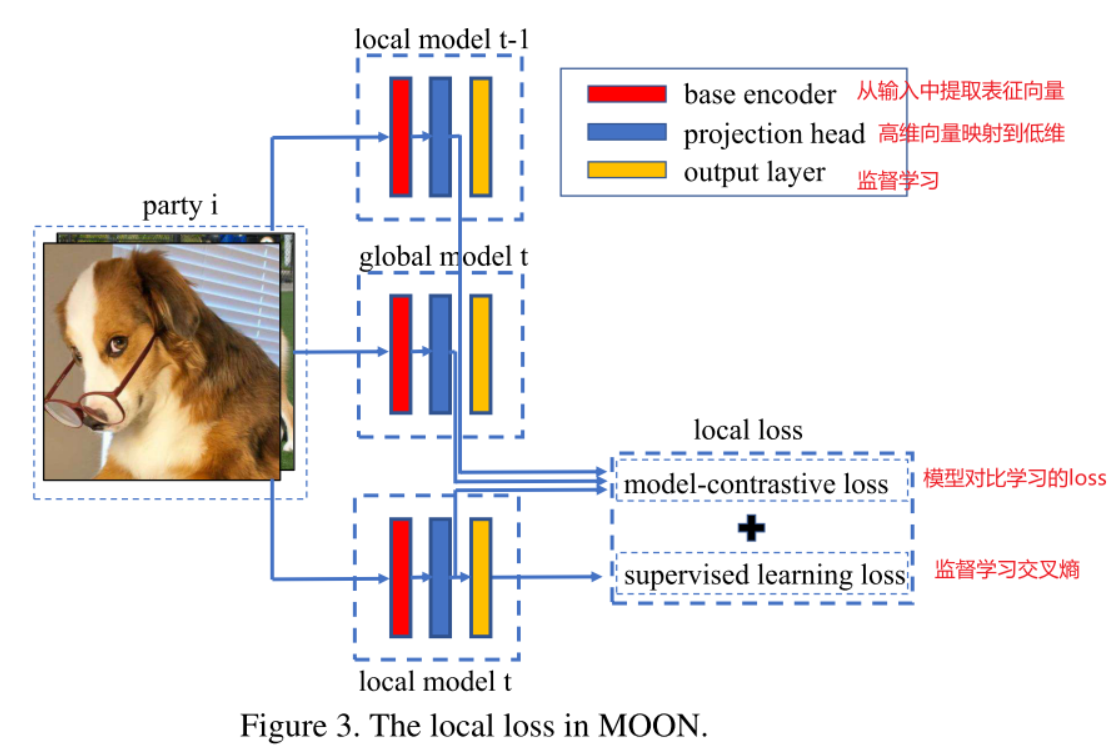The network has three components: a base encoder, a projection head, and an output layer.

## MOON伪代码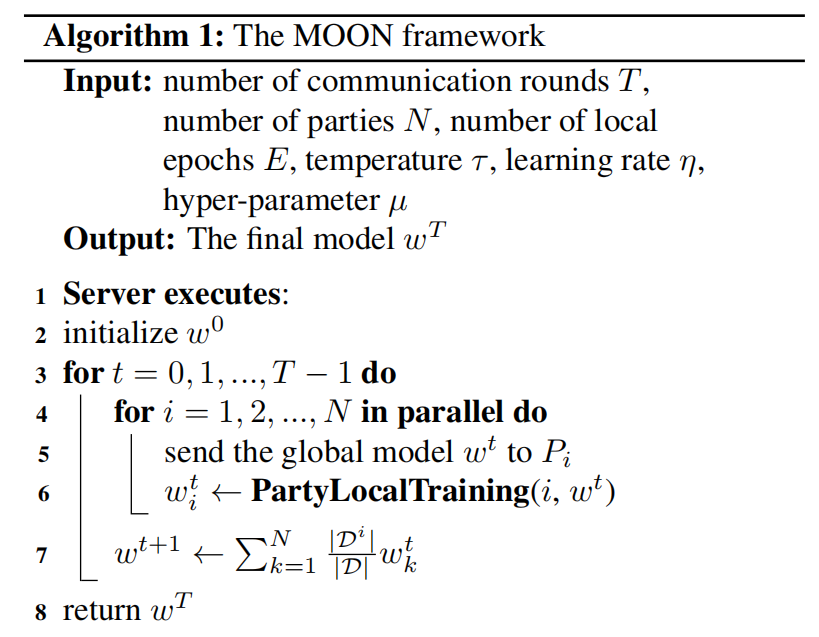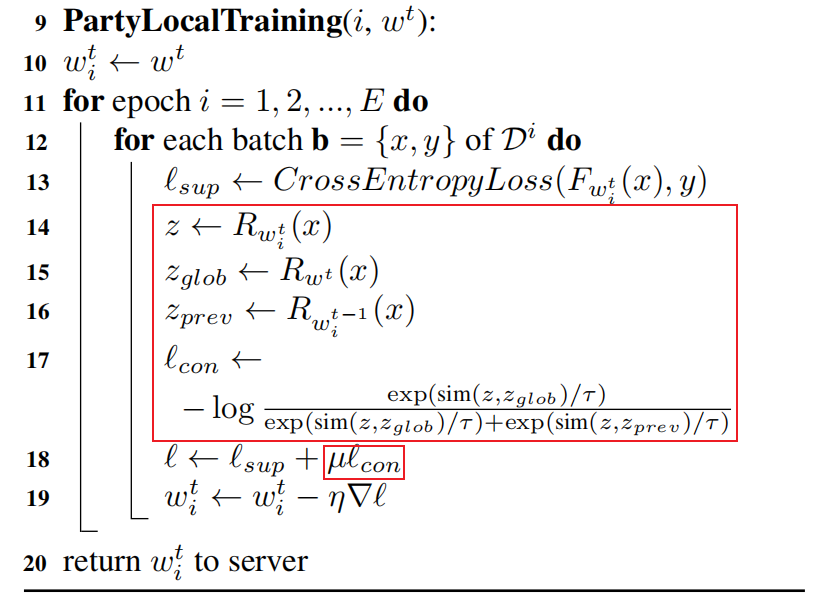## SimCLR和MOON

• SimCLR是想让同一张图片（数据层面）的不同view的表征$z_i$和$z_j$最大程度地相近
• MOON是想让全局模型和本地模型的参数（模型层面）对应的表征$z_{glob}$和$z_{local}$最大程度地相近。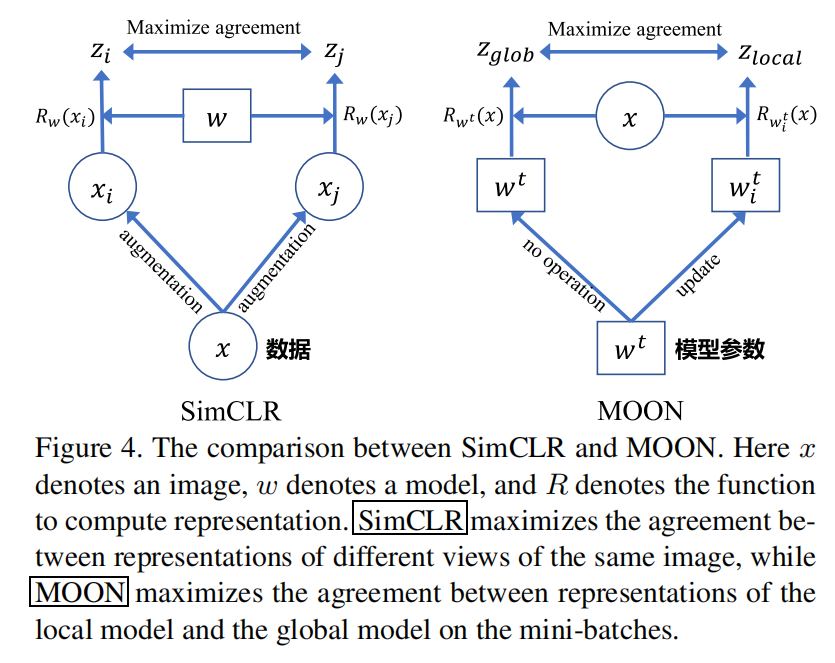# 四、实验

## 数据集

Image classification datasets：CIFAR-10, CIFAR-100, and Tiny-Imagenet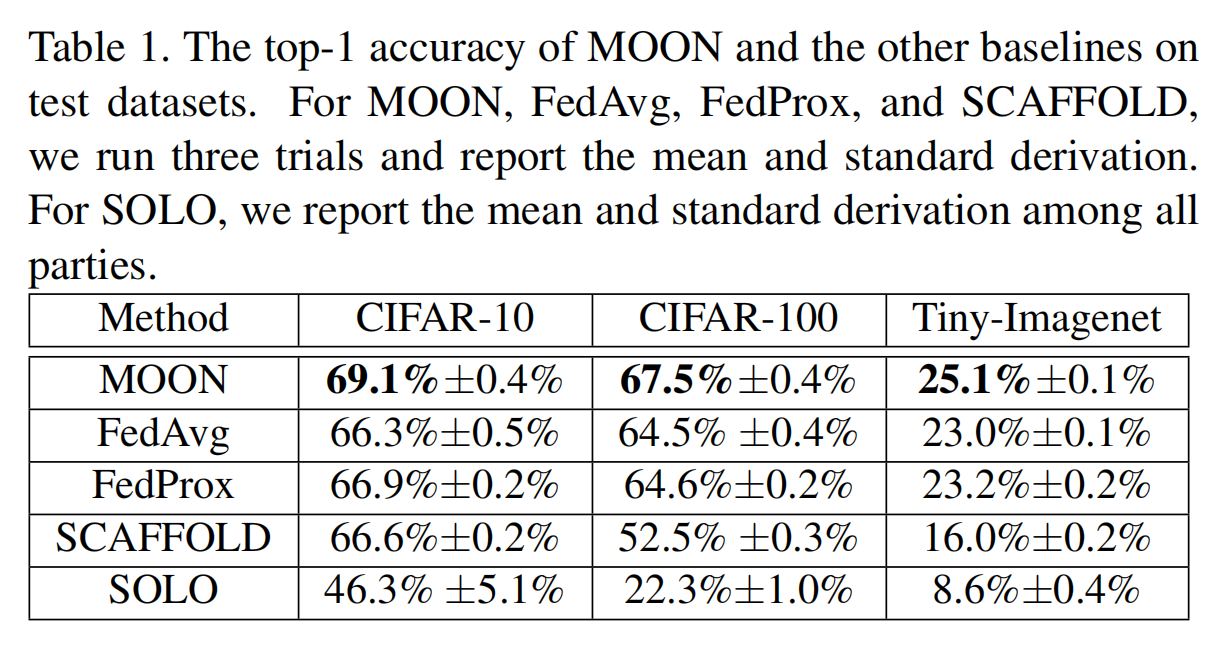SOLO表示每个客户端只利用自己本地数据训练模型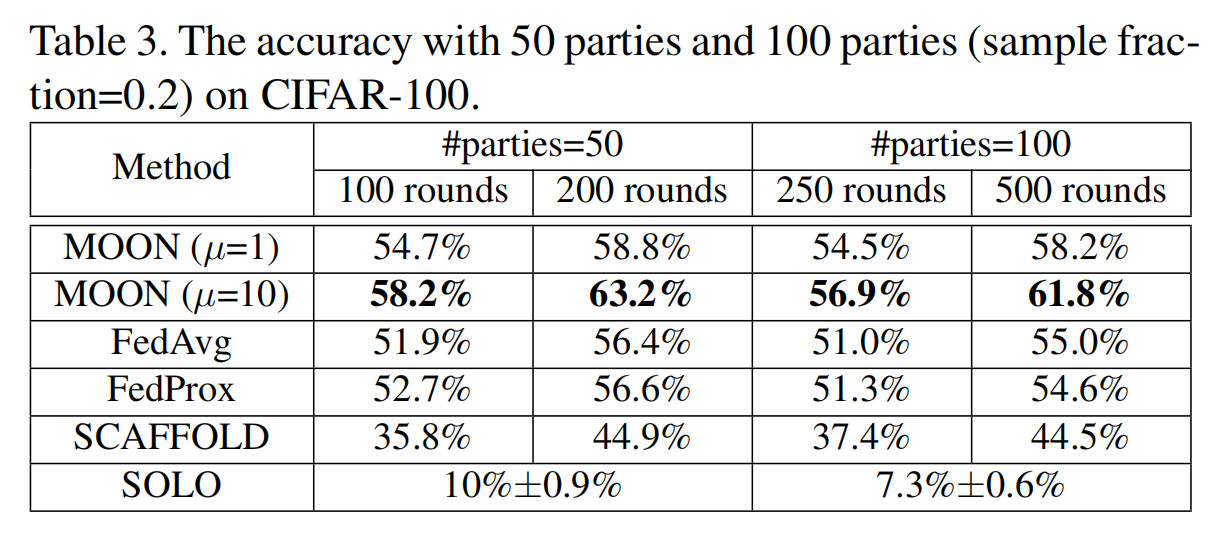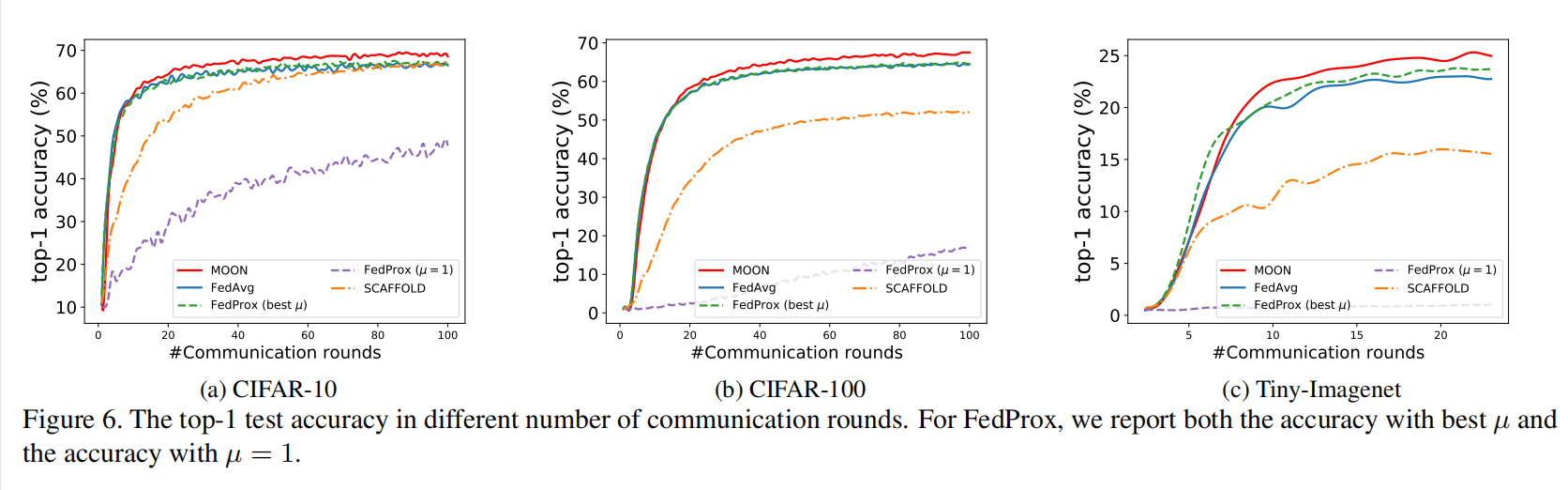# 五、总结

Author:
Reprint policy: All articles in this blog are used except for special statements CC BY 4.0 reprint polocy. If reproduced, please indicate source SHWEI !

Previous【ICML 2018图神经网络论文解读】Representation Learning on Graphs with Jumping Knowledge Networks (JK-Net)

2021-10-19
Next一文读懂BERT模型

2021-10-09
TOC